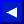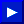EXAMPLES of NOT Operator:
```   10 IF NOT AA = BB AND NOT(BB = CC) THEN...

NN% = NOT 96: PRINT NN%
-97
```

 NOTE: TO find the value of NOT use the expression X=(-(X+1)). (The two's complement of any integer is the bit complement plus one.)

### ON

TYPE: Statement
FORMAT: ON <variable> GOTO / GOSUB <line-number>[,<line-number>]...

Action: The ON statement is used to GOTO one of several given linenumbers, depending upon the value of a variable. The value of the variables can range from zero through the number of lines given. if the value is a non-integer, the fractional portion is left off. For example, if the variable value is 3, ON will GOTO the third line-number in the list.

If the value of the variable is negative, the BASIC error message ?ILLEGAL QUANTITY occurs. If the number is zero, or greater than the number of items in the list, the program just "ignores" the statement and continues with the statement following the ON statement.

ON is really an underused variant of the IF...THEN... statement. Instead of using a whole lot of IF statements each of which sends the program to 1 specific line, 1 ON statement can replace a list of IF statements. When you look at the first example you should notice that the 1 ON statement replaces 4 IF...THEN... statements.

EXAMPLES of ON Statement:
``` ON -(A=7)-2*(A=3)-3*(A<3)-4*(A>7)GOTO 400,900,1000,100
ON X GOTO 100,130,180,220
ON X+3 GOSUB 9000,20,9000
100 ON NUM GOTO 150,300,320,390
500 ON SUM/2 + 1 GOSUB 50,80,20
```This page has been created by Sami Rautiainen. Read the small print. Last updated November 14, 1998.